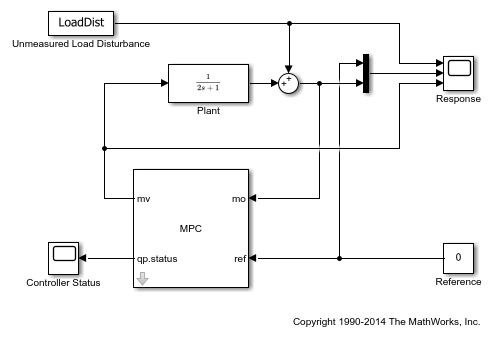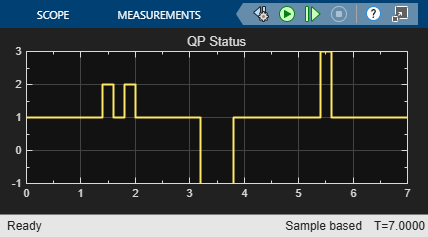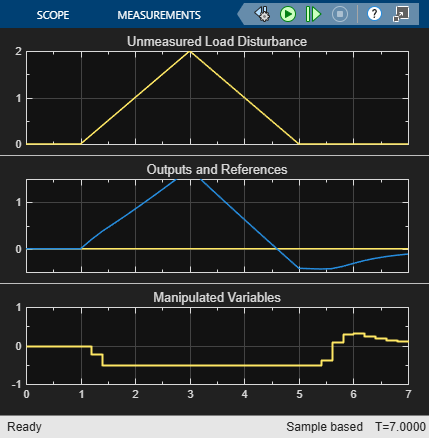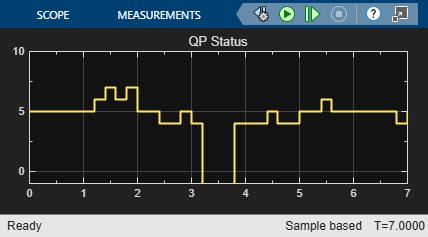# Simulate MPC Controller with a Custom QP Solver

You can simulate the closed-loop response of an MPC controller with a custom quadratic programming (QP) solver in Simulink®.

This example uses an on-line monitoring application, first solving it using the Model Predictive Control Toolbox™ built-in solver, then using a custom solver that uses the `quadprog` (Optimization Toolbox) solver from the Optimization Toolbox™.

Implementing a custom QP solver in this way does not support code generation. For more information on generating code for a custom QP solver, see Simulate and Generate Code for MPC Controller with Custom QP Solver. For more information on QP Solvers, see QP Solvers.

In the on-line monitoring example, the `qp.status` output of the MPC Controller block returns a positive integer whenever the controller obtains a valid solution of the current run-time QP problem and sets the `mv` output. The `qp.status` value corresponds to the number of iterations used to solve this QP.

If the QP is infeasible for a given control interval, the controller fails to find a solution. In that case, the `mv` outport stays at its most recent value and the `qp.status` outport returns `-1`. Similarly, if the maximum number of iterations is reached during optimization (rare), the `mv` outport also freezes and the `qp.status` outport returns `0`.

Real-time MPC applications can detect whether the controller is in a "failure" mode (`0` or `-1`) by monitoring the `qp.status` outport. If a failure occurs, a backup control plan should be activated. This is essential if there is any chance that the QP could become infeasible, because the default action (freezing MVs) may lead to unacceptable system behavior, such as instability. Such a backup plan is, necessarily, application-specific.

### MPC Application with Online Monitoring

The plant used in this example is a single-input, single-output system with hard limits on both the manipulated variable (MV) and the controlled output (OV). The control objective is to hold the OV at a setpoint of `0`. An unmeasured load disturbance is added to the OV. This disturbance is initially a ramp increase. The controller response eventually saturates the MV at its hard limit. Once saturation occurs, the controller can do nothing more, and the disturbance eventually drives the OV above its specified hard upper limit. When the controller predicts that it is impossible to force the OV below this upper limit, the run-time QP becomes infeasible.

Define the plant as a first-order SISO system with unity gain.

```Plant = tf(1,[2 1]); ```

Define the unmeasured load disturbance. The signal ramps up from `0` to `2` between `1` and `3` seconds, then ramps back down from `2` to `0` between `3` and `5` seconds.

```LoadDist = [0 0; 1 0; 3 2; 5 0; 7 0]; ```

### Design MPC Controller

Create an MPC object using the model of the test plant. The chosen control interval is about one tenth of the dominant plant time constant.

```Ts = 0.2; mpcobj = mpc(Plant, Ts); ```
```-->"PredictionHorizon" is empty. Assuming default 10. -->"ControlHorizon" is empty. Assuming default 2. -->"Weights.ManipulatedVariables" is empty. Assuming default 0.00000. -->"Weights.ManipulatedVariablesRate" is empty. Assuming default 0.10000. -->"Weights.OutputVariables" is empty. Assuming default 1.00000. ```

Define hard constraints on the plant input (MV) and output (OV). By default, all the MV constraints are hard and OV constraints are soft.

```mpcobj.MV.Min = -0.5; mpcobj.MV.Max = 1; mpcobj.OV.Min = -1; mpcobj.OV.Max = 1; mpcobj.OV.MinECR = 0; % change OV lower limit from soft to hard mpcobj.OV.MaxECR = 0; % change OV upper limit from soft to hard ```

Generally, hard OV constraints are discouraged and are used here only to illustrate how to detect an infeasible QP. Hard OV constraints make infeasibility likely, in which case a backup control plan is essential. This example does not include a backup plan. However, as shown in the simulation, the default action of freezing the single MV is the best response in this simple case.

### Simulate Using Simulink with Built-in QP Solver

```% Build the control system in a Simulink model and enable the |qp.status| % outport by selecting the *Optimization status* parameter of the MPC % Controller block. Display the run-time |qp.status| value in the % Controller Status scope. mdl = 'mpc_onlinemonitoring'; open_system(mdl) ```Simulate the closed-loop response using the default Model Predictive Control Toolbox QP solver.

```open_system([mdl '/Controller Status']) open_system([mdl '/Response']) sim(mdl) ```
```-->Converting the "Model.Plant" property to state-space. -->Converting model to discrete time. -->Assuming output disturbance added to measured output #1 is integrated white noise. -->"Model.Noise" is empty. Assuming white noise on each measured output. ```### Explanation of the Closed-Loop Response

As shown in the response scope, at 1.4 seconds, the increasing disturbance causes the MV to saturate at its lower bound of -0.5, which is the QP solution under these conditions (because the controller is trying to hold the OV at its setpoint of `0`).

The OV continues to increase due to the ramp disturbance and, at 2.2 seconds, exceeds the specified hard upper bound of `1.0`. Since the QP is formulated in terms of predicted outputs, the controller still predicts that it can bring OV back below 1.0 in the next move and therefore the QP problem is still feasible.

Finally, at t = 3.2 seconds, the controller predicts that it can no longer move the OV below 1.0 within the next control interval, and the QP problem becomes infeasible and `qp.status` changes to -1 at this time.

After three seconds, the disturbance is decreasing. At 3.8 seconds, the QP becomes feasible again. The OV is still well above its setpoint, however, and the MV remains saturated until 5.4 seconds, when the QP solution is to increase the MV as shown. From then on, the MV is not saturated, and the controller is able to drive the OV back to its setpoint.

When the QP is feasible, the built-in solver finds the solution in three iterations or less.

### Simulate with a Custom QP Solver

To examine how the custom solver behaves under the same conditions, activate the custom solver option by setting the `Optimizer.CustomSolver` property of the MPC controller.

```mpcobj.Optimizer.CustomSolver = true; ```

You must also provide a MATLAB® function that satisfies all the following requirements:

• Function name must be `mpcCustomSolver`.

• Function input and output arguments must match those defined in the `mpcCustomSolver.txt` template file.

• Function must be on the MATLAB path.

For this example, use the custom solver defined in `mpcCustomSolver.txt`, which uses the `quadprog` command from the Optimization Toolbox as the custom QP solver. To implement your own custom QP solver, modify this file.

Save the function in your working folder as a `.m` file.

```src = which('mpcCustomSolver.txt'); dest = fullfile(pwd,'mpcCustomSolver.m'); copyfile(src,dest,'f'); ```

Review the saved `mpcCustomSolver.m` file.

```function [x, status] = mpcCustomSolver(H, f, A, b, x0) % mpcCustomSolver allows user to specify a custom quadratic programming % (QP) solver to solve the QP problem formulated by MPC controller. When % the "mpcobj.Optimizer.CustomSolver" property is set true, instead of % using the built-in QP solver, MPC controller will now use the customer QP % solver defined in this function for simulations in MATLAB and Simulink. % % The MPC QP problem is defined as follows: % Find an optimal solution, x, that minimizes the quadratic objective % function, J = 0.5*x'*H*x + f'*x, subject to linear inequality % constraints, A*x >= b. % % Inputs (provided by MPC controller at run-time): % H: a n-by-n Hessian matrix, which is symmetric and positive definite. % f: a n-by-1 column vector. % A: a m-by-n matrix of inequality constraint coefficients. % b: a m-by-1 vector of the right-hand side of inequality constraints. % x0: a n-by-1 vector of the initial guess of the optimal solution. % % Outputs (fed back to MPC controller at run-time): % x: must be a n-by-1 vector of optimal solution. % status: must be an finite integer of: % positive value: number of iterations used in computation % 0: maximum number of iterations reached % -1: QP is infeasible % -2: Failed to find a solution due to other reasons % Note that even if solver failed to find an optimal solution, "x" must be % returned as a n-by-1 vector (i.e. set it to the initial guess x0) % % DO NOT CHANGE LINES ABOVE % The following code is an example of how to implement the custom QP solver % in this function. It requires Optimization Toolbox to run. % Define QUADPROG options and turn off display of optimization results in % Command window. options = optimoptions('quadprog'); options.Display = 'none'; % By definition, constraints required by "quadprog" solver is defined as % A*x <= b. However, in our MPC QP problem, the constraints are defined as % A*x >= b. Therefore, we need to implement some conversion here: A_custom = -A; b_custom = -b; % Compute the QP's optimal solution. Note that the default algorithm used % by "quadprog" ('interior-point-convex') ignores x0. "x0" is used here as % an input argument for illustration only. H = (H+H')/2; % ensure Hessian is symmetric [x, ~, Flag, Output] = quadprog(H, f, A_custom, b_custom, [], [], [], [], x0, options); % Converts the "flag" output to "status" required by the MPC controller. switch Flag case 1 status = Output.iterations; case 0 status = 0; case -2 status = -1; otherwise status = -2; end % Always return a non-empty x of the correct size. When the solver fails, % one convenient solution is to set x to the initial guess. if status <= 0 x = x0; end ```

Repeat the simulation.

```set_param([mdl '/Controller Status'],'ymax','10'); sim(mdl) ```
```-->Converting the "Model.Plant" property to state-space. -->Converting model to discrete time. -->Assuming output disturbance added to measured output #1 is integrated white noise. -->"Model.Noise" is empty. Assuming white noise on each measured output. ```The plant input and output signals are identical to those obtained using the built-in Model Predictive Control Toolbox solver, but the `qp.status` shows that `quadprog` does not take the same number of iterations to find a solution. However, it does detect the same infeasibility time period.

```bdclose(mdl) ```